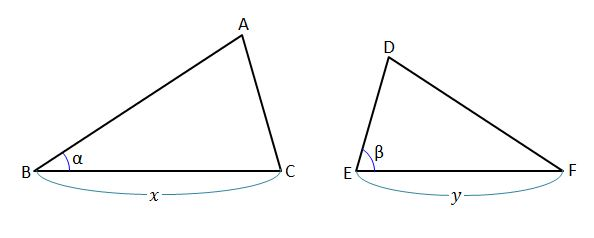# What Makes These Similar?

Geometry Level 1In the above diagram, $x=21,$ $y=14,$ $\alpha={35}^\circ,$ and $\beta={75}^\circ.$ Which condition would mean that the two triangles are similar?

Note: The above diagram is not drawn to scale.

×